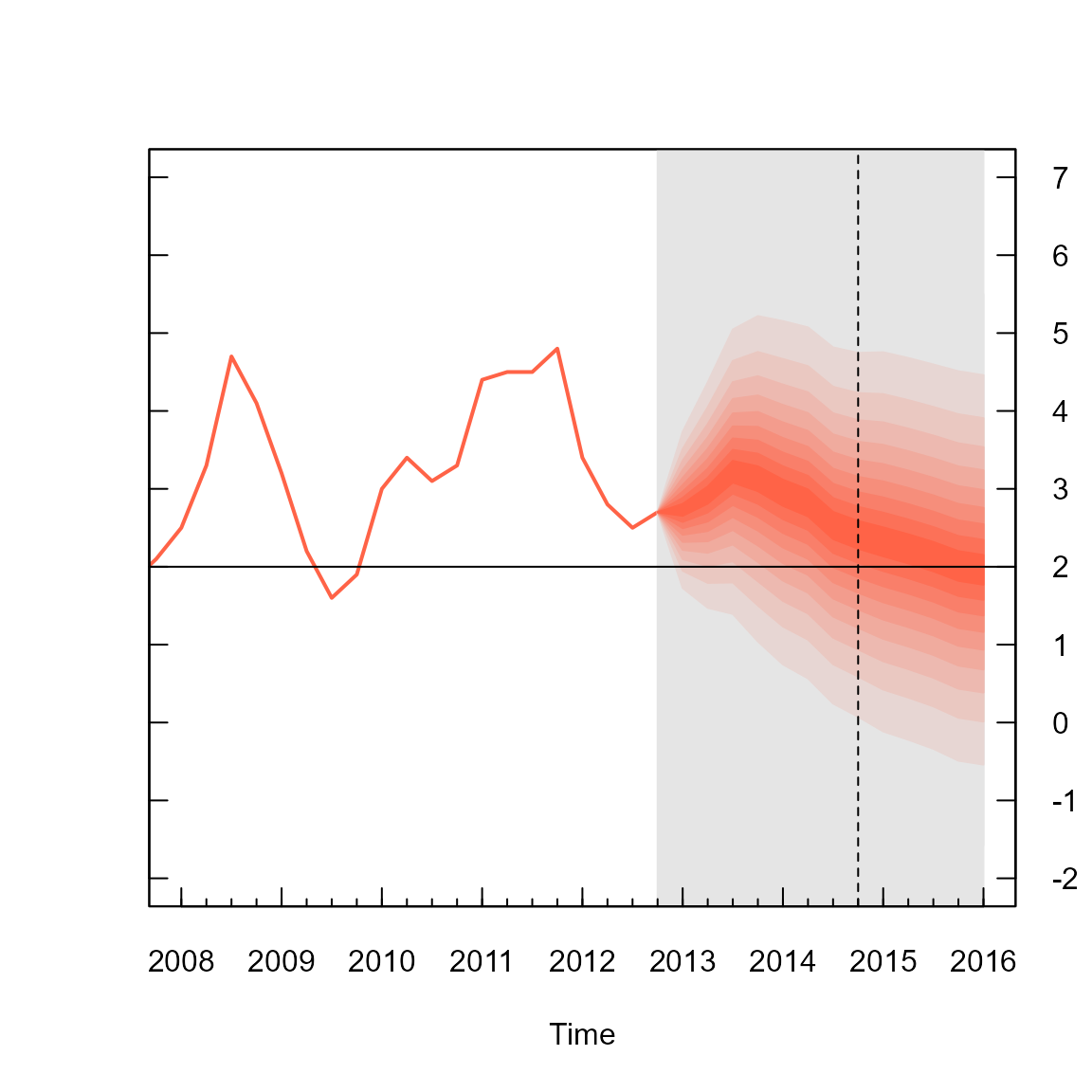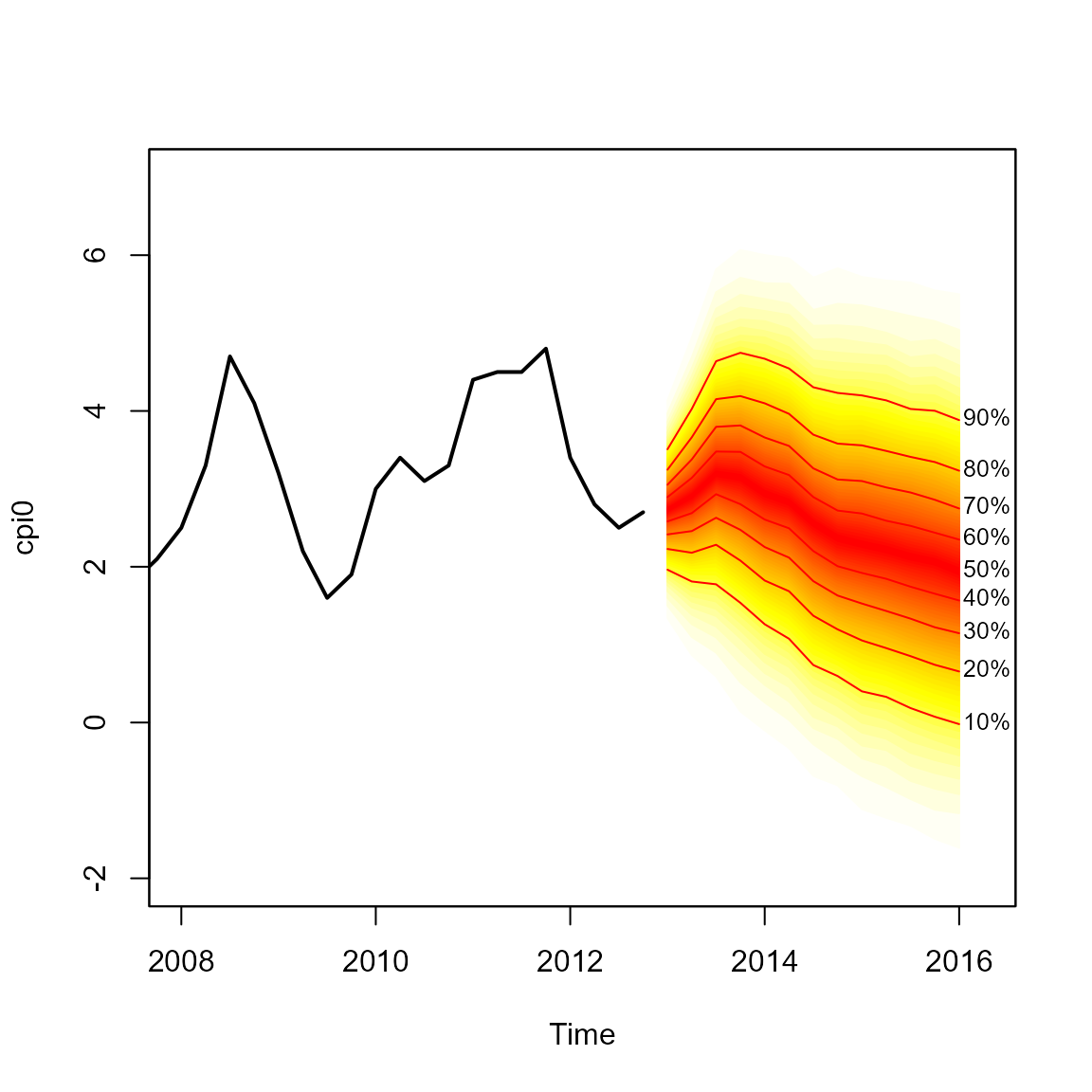The Bank of England were one of the earliest pioneers of using fan charts to illustrate uncertainty in their forecasts, similar to this one:Bank of England February 2013 CPI Fan Chart. Obtained from Chart 5.3 in February 2013 Inflation Report

As far as I can tell these charts were made in Excel, although (appropriately) I am not completely certain. There are also MATLAB scripts to create fan charts.

The fanplot package can also replicate Bank of England fan charts in R….

## Split Normal (Two-Piece) Normal Distribution.

The Bank of England produce fan charts of forecasts for CPI and GDP in their quarterly Inflation Reports. They also provide data, in the form of mode, uncertainty and a skewness parameters of a split-normal distribution that underlie their fan charts (The Bank of England predominately refer to the equivalent, re-parametrised, two-piece normal distribution). The probability density of the split-normal distribution is given by Julio (2007) as

$f(x; \mu, \sigma_1, \sigma_2) = \left\{\begin{array}{ll}\frac{\sqrt 2}{\sqrt\pi (\sigma_1+\sigma_2)} e^{-\frac{1}{2\sigma_1^2}(x-\mu)^2} \quad \mbox{for } -\infty < x \leq \mu \\\frac{\sqrt 2}{\sqrt\pi (\sigma_1+\sigma_2)} e^{-\frac{1}{2\sigma_2^2}(x-\mu)^2} \quad \mbox{for } \mu < x < \infty \\\end{array},\right.$

where $$\mu$$ represents the mode parameter, and the two standard deviations $$\sigma_1$$ and $$\sigma_2$$ can be derived given the overall uncertainty parameter, $$\sigma$$ and skewness parameters, $$\gamma$$, as; $$\sigma^2=\sigma^2_1(1+\gamma)=\sigma^2_2(1-\gamma).$$ The fanplot package contains functions for the density, distribution and quantile of a split normal distribution (dsplitnorm, psplitnorm and qsplitnorm) and a random generator function rsplitnorm

## Fan Chart Plots for CPI.

In order to reproduce the Bank of England plots there are two data sets in the fanplot package. The cpi object is a time series data frame with past values of CPI index. The boe object is a data frame with historical details on the split normal parameters for CPI inflation between Q1 2004 to Q4 2013 forecasts published by the Bank of England.

library(fanplot)
head(boe)
##   time0    time mode uncertainty skew
## 1  2004 2004.00 1.34      0.2249    0
## 2  2004 2004.25 1.60      0.3149    0
## 3  2004 2004.50 1.60      0.3824    0
## 4  2004 2004.75 1.71      0.4274    0
## 5  2004 2005.00 1.77      0.4499    0
## 6  2004 2005.25 1.68      0.4761    0

The first column time0 refers to the base year of forecast, the second, time indexes future projections, whilst the remaining three columns provide values for the corresponding projected mode ($$\mu$$), uncertainty ($$\sigma$$) and skew ($$\gamma$$) parameters: Users can replicate past Bank of England fan charts for a particular period after creating a matrix object that contains values on the split-normal quantile function for a set of user defined probabilities. For example, in the code below, a subset of the Bank of England future parameters of CPI published in Q1 2013 are first selected. Then a vector of probabilities related to the percentiles, that we ultimately would like to plot different shaded fans for, are created. Finally, in a for loop the qsplitnorm function, calculates the values for which the time-specific (i) split-normal distribution will be less than or equal to the probabilities of p.

# select relevant data
y0 <- 2013
boe0 <- subset(boe, time0==y0)
k <- nrow(boe0)

# guess work to set percentiles the BOE are plotting
p <- seq(0.05, 0.95, 0.05)
p <- c(0.01, p, 0.99)

# quantiles of split-normal distribution for each probability
# (row) at each future time point (column)
cpival <- matrix(NA, nrow = length(p), ncol = k)
for (i in 1:k)
cpival[, i] <- qsplitnorm(p, mode = boe0$mode[i], sd = boe0$uncertainty[i], skew = boe0$skew[i]) The new object cpival contains the values evaluated from the qsplitnormfunction in 6 rows and 13 columns, where rows represent the probabilities used in the calculation p and columns represent successive time periods. cpival[1:5, 1:5] ## [,1] [,2] [,3] [,4] [,5] ## [1,] 1.310928 0.8728139 0.6377539 0.1755382 -0.1673062 ## [2,] 1.726639 1.4725288 1.3942125 1.0410359 0.7458961 ## [3,] 1.948254 1.7922346 1.7974778 1.5024295 1.2327209 ## [4,] 2.097776 2.0079386 2.0695589 1.8137296 1.5611793 ## [5,] 2.216611 2.1793733 2.2858004 2.0611410 1.8222275 The object cpival can be used to add a fan chart to the active R graphic device. In the code below, the area of the plot is set up when plotting the past CPI data, contained in the time series object cpi. The xlim arguments are set to ensure space on the right hand side of the plotting area for the fan. Following the Bank of England style for plotting fan charts, the background for future values is set to a gray colour, y-axis are plotted on the right hand side, a horizontal line are added for the CPI target and a vertical line for the two-year ahead point. # past data plot(cpi, type = "l", col = "tomato", lwd = 2, xlim = c(y0 - 5, y0 + 3), ylim = c(-2, 7), xaxt = "n", yaxt = "n", ylab="") # background rect(y0 - 0.25, par("usr") - 1, y0 + 3, par("usr") + 1, border = "gray90", col = "gray90") # add fan fan(data = cpival, data.type = "values", probs = p, start = y0, frequency = 4, anchor = cpi[time(cpi) == y0 - 0.25], fan.col = colorRampPalette(c("tomato", "gray90")), ln = NULL, rlab = NULL) # boe aesthetics axis(2, at = -2:7, las = 2, tcl = 0.5, labels = FALSE) axis(4, at = -2:7, las = 2, tcl = 0.5) axis(1, at = 2008:2016, tcl = 0.5) axis(1, at = seq(2008, 2016, 0.25), labels = FALSE, tcl = 0.2) abline(h = 2) #boe cpi target abline(v = y0 + 1.75, lty = 2) #2 year lineThe fan chart itself is outputted from the fan function, where arguments are set to ensure a close resemblance of the R plot to that produced by the Bank of England. The first three arguments in the fan function called in the above code, provide the cpival data to plotted, indicate that the data are a set of calculated values (as opposed to simulations) and provide the probabilities that correspond to each row of cpival object. The next two arguments define the start time and frequency of the data. These operate in a similar fashion to those used when defining time series in R with the ts function. The anchor argument is set to the value of CPI before the start of the fan chart. This allows a join between the value of the Q1 2013 observation and the fan chart. The fan.col argument is set to a colour palette for shades between tomato and gray90. The final two arguments are set to NULL to suppress the plotting of contour lines at the boundary of each shaded fan and their labels, as per the Bank of England style. ## Default Fan Chart Plot. By default, the fan function treats objects passed to the data argument as simulations from sequential distributions, rather than user-created values corresponding probabilities provided in the probs argument (as above). An alternative plot below, based on simulated data and default style settings in the fan function produces a fan chart with a greater array of coloured fans with labels and contour lines alongside selected percentiles of the future distribution. To illustrate we can simulate 10,000 values from the future split-normal distribution parameters from Q1 2013 in the boe0 data frame using the rsplitnormfunction #simulate future values cpisim <- matrix(NA, nrow = 10000, ncol = k) for (i in 1:k) cpisim[, i] <- rsplitnorm(n = 10000, mode = boe0$mode[i], sd = boe0$uncertainty[i], skew = boe0$skew[i])

The fan chart based on the simulations in cpisim can then be added to the plot;

# truncate cpi series
cpi0 <- ts(cpi[time(cpi)<2013], start=start(cpi), frequency=frequency(cpi) )

# past data
plot(cpi0, type = "l", lwd = 2, xlim = c(y0 - 5, y0 + 3.25), ylim = c(-2, 7))

fan(data = cpisim, start = y0, frequency = 4)The fan function calculates the values of 100 equally spaced percentiles of each future distribution when the default data.type = "simulations" is set. This allows 50 fans to be plotted from the heat.colours colour palate, providing a finer level of shading in the representation of future distributions. In addition, lines and labels are provided along each decile. The fan chart does not connect to the last observation as anchor = NULL by default.